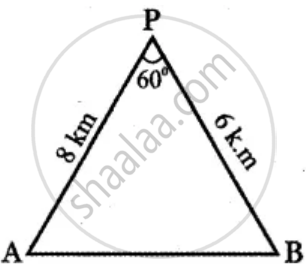Tamil Nadu Board of Secondary EducationHSC Arts Class 11th

# A researcher wants to determine the width of a pond from east to west, which cannot be done by actual measurement. From a point P, he finds the distance to the eastern-most point of the pond to be - Mathematics

Sum

A researcher wants to determine the width of a pond from east to west, which cannot be done by actual measurement. From a point P, he finds the distance to the eastern-most point of the pond to be 8 km, while the distance to the westernmost point from P to be 6 km. If the angle between the two lines of sight is 60°, find the width of the pond

#### SolutionA – be the easternmost point on the pond and

B – be the westernmost point on the pond.

AB – Width of the pond

P – Point of observation.

The distance of A from P = 8 km

Distance of B from P = 6 km

Angle between the directions PA and PB

∠APB = 60°

In ∆PAB,

Using cosine formula

AB2 = PA2 + PB2 – 2PA . PB . cos∠APB

AB2 = 82 + 62 – 2 × 8 × 6 . cos 60°

= 64 xx 36 - 96 xx 1/2

= 100 – 48

= 52

AB = sqrt(52)

= sqrt(4 xx 13)

AB = 2sqrt(13) k.m.

Width of the pond = 2sqrt(13) k.m.

Concept: Application to Triangle
Is there an error in this question or solution?

Share